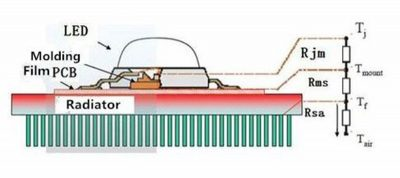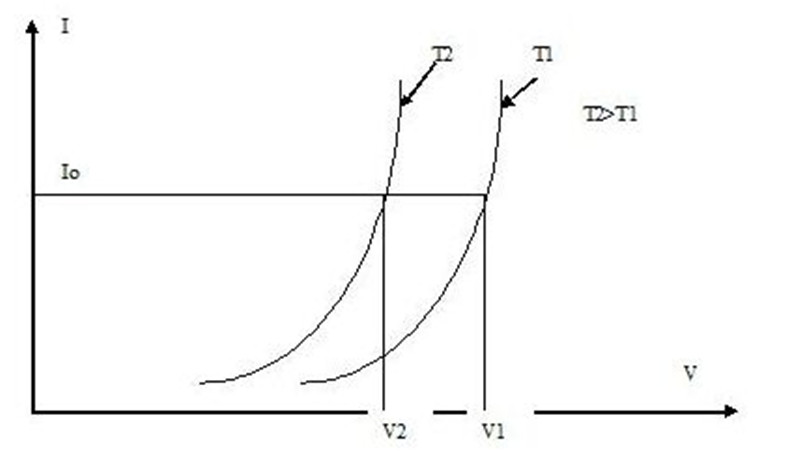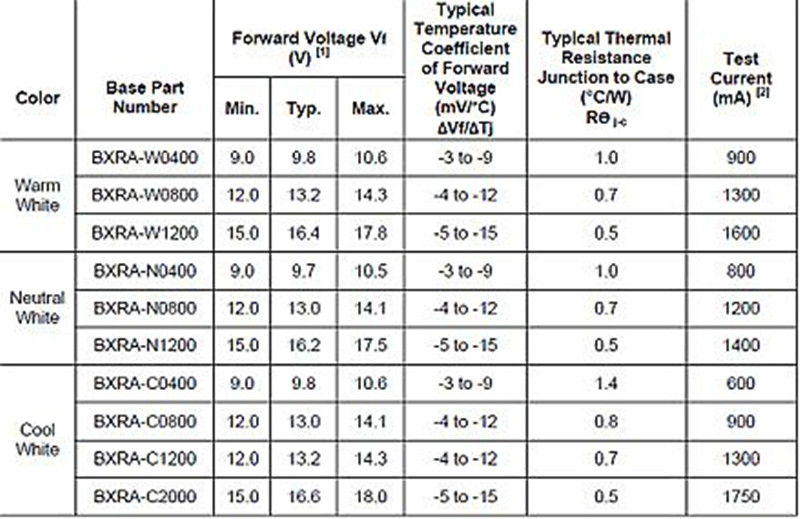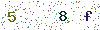﻿ How to Test the Junction Temperature of LED? - LISUNLISUN

# How to Test the Junction Temperature of LED?

We know that the life of LED is closely related to its junction temperature. The key to prolonging its life is to reduce its junction temperature. And the key to reduce the junction temperature is to have a good heat sink that able to exhaust the heat generated by LED in time.

So, how to measure the junction temperature of LED?

Junction temperature seems to be a temperature measurement problem, but the junction temperature need to measure is in the internal of LED. Can’t we measure its temperature with a thermometer or a thermocouple into the PN junction. Of course, its shell temperature can be measured with thermocouple, and then its junction temperature can be calculated according to the thermal resistance Rjc (junction to the shell).

But things become more complicated after installing the radiator. Because usually LED is welded to the aluminum substrate, and the aluminum substrate is installed on the radiator. If you can only measure the temperature of the radiator shell, then it is necessary to know the value of a lot of thermal resistance to calculate the junction temperature.

Including Rjc (junction to case), Rcm (shell to the aluminum substrate, in fact, which also should include film printed version of the thermal resistance), Rms (aluminum substrate to the radiator), Rsa (heat sink to air), which only with one inaccurate data will affect the accuracy of the test. Figure 1 shows a schematic diagram of the LED to each thermal resistance of radiator. Where incorporates a lot of thermal resistance, making it more precision limited. That is to say, the accuracy of the junction temperature would be worse when inferred from the surface temperature of the radiator.Fortunately, there is an indirect method of measuring temperature that is to measure the voltage. Then the junction temperature is relative to which voltage? And what is the relationship?
Firstly, we should talk about the characteristics of LED volt-ampere.
We know that the LED is a semiconductor diode, which has a volt-ampere characteristic same as all diodes. The volt ampere characteristic has a temperature characteristic that is when the temperature raises, the volt ampere characteristic left shifts. The Figure. 2. shows temperature characteristics of the LED’s volt ampere.Figure-2-Temperature-characteristics-of-LED-volt-ampere

Assuming that the LED is powered by a Io constant current, when the voltage is T1, the voltage is V1. While the junction temperature rises to T2, the entire volt ampere characteristic is left shifted, the current Io is constant, the voltage changes to V2. The two voltage difference is removed by temperature, then the temperature coefficient can be obtained and shown by mV/oC. For ordinary silicon diodes, the temperature coefficient is about -2mV/oC.

But the LED is not made of silicon material, so its temperature coefficient should also be measured additionally. Fortunately, most of the LED manufacturers give its temperature coefficient in the data table. Take XLamp7090XR-E high power LED for example, its temperature coefficient is -4mV/oC, and 2 times larger than the ordinary silicon diode. The volt ampere characteristic temperature coefficient of Luxeon Rebel from a company of USA is -2 – 4mV/oC. As for LED Puri array (BXRA) of the USA, it gives more detailed data.Figure-3-Decay-curves-of-LuxeonK2

However, the range of the data is too large, so that may loss the value of the using. Anyway, as long as the temperature coefficient of the LED is known, the LED junction temperature can be calculated from the forward voltage of the LED.

Lisun developed the T3 LED junction temperature thermal resistance electrical characteristic test, it can automatically record the changes of the parameters with time curve.

Lisun Instruments Limited was found by LISUN GROUP in 2003. LISUN quality system has been strictly certified by ISO9001:2015. As a CIE Membership, LISUN products are designed based on CIE, IEC and other international or national standards. All products passed CE certificate and authenticated by the third party lab.